Foxtable(狐表)用户栏目专家坐堂 → [求助]生僻字

共有419人关注过本帖树形打印复制链接

# 主题：[求助]生僻字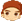michl
1楼 | 信息 | 搜索 | 邮箱 | 主页 | UC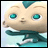加好友发短信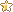[求助]生僻字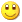Post By：2022/1/18 21:42:00 [只看该作者]

 生僻字占了字符位 但是显示不出来怎么办'''If e.DataCol.Name = "示例" And e.DataRow.IsNull("示例") = False Then    e.DataRow("取左2") = left(e.DataRow("示例"),2)End If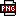此主题相关图片如下：无标题.png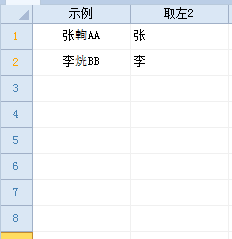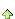2楼 | 信息 | 搜索 | 邮箱 | 主页 | UC加好友发短信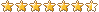Post By：2022/1/18 22:19:00 [只看该作者]

 我测试可以，请上传实例说明michl
3楼 | 信息 | 搜索 | 邮箱 | 主页 | UC加好友发短信Post By：2022/1/19 8:59:00 [只看该作者]

 下载信息  [文件大小：   下载次数： ]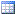点击浏览该文件:示例.table4楼 | 信息 | 搜索 | 邮箱 | 主页 | UC加好友发短信Post By：2022/1/19 9:31:00 [只看该作者]

 这种一个字符居然占2个长度，这样可以： e.DataRow("取左2") = left(e.DataRow("示例"),3)但是普通字符就有问题了，网上搜了一圈，没有找到合适的方法michl
5楼 | 信息 | 搜索 | 邮箱 | 主页 | UC加好友发短信Post By：2022/1/19 9:41:00 [只看该作者]

 呃…那就先这样空白着吧。取3位不行的，影响大量普通字符6楼 | 信息 | 搜索 | 邮箱 | 主页 | UC加好友发短信Post By：2022/1/19 9:55:00 [只看该作者]

 试试这样处理一下If e.DataCol.Name = "示例" And e.DataRow.IsNull("示例") = False Then    e.DataRow("取左2") = left(e.DataRow("示例"),2)    Dim bs() As Byte = System.Text.Encoding.GetEncoding("GB2312").GetBytes(e.DataRow("取左2"))    If bs(bs.length - 1) = 63 Then        e.DataRow("取左2") = left(e.DataRow("示例"),3)    End IfEnd Ifmichl
7楼 | 信息 | 搜索 | 邮箱 | 主页 | UC加好友发短信Post By：2022/1/19 11:15:00 [只看该作者]

 可以取出的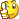用在select里也试了一下，同样正确！If e.DataCol.Name = "示例" And e.DataRow.IsNull("示例") = False Then    Dim s1 = left(e.DataRow("示例"),2)    Dim drs1 As List(of DataRow) = DataTables("表A").Select("示例 like'" & s1 & "*'")    e.DataRow("取左2") = s1    e.DataRow("左2同数") = drs1.Count    Dim bs() As Byte = System.Text.Encoding.GetEncoding("GB2312").GetBytes(e.DataRow("取左2"))    If bs(bs.length - 1) = 63 Then        Dim s2 = left(e.DataRow("示例"),3)        Dim drs2 As List(of DataRow) = DataTables("表A").Select("示例 like'" & s2 & "*'")        e.DataRow("取左2") = s2        e.DataRow("左2同数") = drs2.Count    End IfEnd If8楼 | 信息 | 搜索 | 邮箱 | 主页 | UC加好友发短信Post By：2022/1/19 11:22:00 [只看该作者]

 可以优化一下If e.DataCol.Name = "示例" And e.DataRow.IsNull("示例") = False Then    Dim s1 = left(e.DataRow("示例"),2)    Dim bs() As Byte = System.Text.Encoding.GetEncoding("GB2312").GetBytes(s1)    If bs(bs.length - 1) = 63 Then        s1 = left(e.DataRow("示例"),3)    End If    Dim drs1 As List(of DataRow) = DataTables("表A").Select("示例 like'" & s1 & "*'")    e.DataRow("取左2") = s1    e.DataRow("左2同数") = drs1.CountEnd Ifmichl
9楼 | 信息 | 搜索 | 邮箱 | 主页 | UC加好友发短信Post By：2022/1/19 11:50:00 [只看该作者]

 好的谢谢